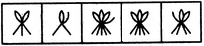# Non Verbal Reasoning - Classification - Discussion

Discussion Forum : Classification - Section 2 (Q.No. 3)
Directions to Solve

In each problem, out of the five figures marked (1), (2), (3), (4) and (5), four are similar in a certain manner. However, one figure is not like the other four. Choose the figure which is different from the rest.

3.

Choose the figure which is different from the rest.(1)     (2)     (3)     (4)     (5)
1
2
3
4
5
Explanation:
In each one of the figurers except fig. (3), the number of half-leaves is one more than the number of line segments.
Discussion:
8 comments Page 1 of 1.

Mamidala said:   3 months ago
In the first figure there are 4 half leaves and lines 3 (difference is one)
In 2nd fig, there are 3 half leaves and 2 lines(different is one)
In 3rd fig, there are 7 half leaves and 5 lines (difference two)
In 4th one, there are 6 half leaves and 5 lines (difference is one )
In 5th fig, there are 5 half leaves and 4 lines (difference is one)
So, option 3 is the correct answer.

Vidhi said:   4 years ago
Line segments are in odd number in all, except 3, in it even 6 line segments are there.

Harishvivek said:   5 years ago
1 and 2 possible:

a) 1 as the middle leaf is missing.
b) 2 as it does not have 2 complete leaves.
c) most number of half-leaves but sounds logically incorrect.

Brizzler said:   6 years ago
Number 1 has 4 half leaves and 3 line segment.
Number 2 has 3 half leaves and 2 line segment.
Number 3 has 7 half leaves and 6 line segment.
Number 4 has 6 half leaves and 6 line segment.
Number 5 has 5 half leaves and 4 line segment.

So answer should be option D not C.
(1)

Dandy said:   6 years ago
Number 5, only one where leaf is pointing to the left.

Abc said:   7 years ago
We have 1 full and one-half leave, 2 full and 1 half leaf, which is equal to 2 & 3 full leaves respectively. So option 3 is the diffrent from rest.

Abc said:   7 years ago
According to my logic we can also take option 1 as an answer which has the least leaves.

EFK Shanpoov said:   7 years ago
Figs 1&4 are don't have a half leaves so leave it.

Remain 3 figs have a half leaves. Then which one have a more leaves with half leaves with in these three leaves so pic fig 3. Tats the answer.

Simple logic is which one have max leaves.